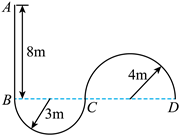$\text{A.}$ $t=\left(2+\frac{7 \pi}{4}\right) \mathrm{s}, l=8 \mathrm{~m}$ $\text{B.}$ $t=\left(\frac{9}{4}+\frac{7 \pi}{2}\right) \mathrm{s}, l=5 \mathrm{~m}$. $\text{C.}$ $t=\left(2+\frac{5}{12} \sqrt{6}+\frac{7 \sqrt{6} \pi}{6}\right) \mathrm{s}, l=5.5 \mathrm{~m}$. $\text{D.}$ $t=\left[2+\frac{5}{12} \sqrt{6}+\frac{(\sqrt{6}+4) \pi}{2}\right] \mathrm{s}, l=5.5 \mathrm{~m}$

B

#### 解析：

$$v_{2 \mathrm{~m}}=2 \mathrm{~m} / \mathrm{s} < v_{1 \mathrm{~m}}$$

$A B$ 段从最大速度 $1 \mathrm{~m}$ 减速到 $v$ 的时间 $t_1=\frac{v_{\mathrm{m}}-v}{a_1}=\frac{4-2}{2} \mathrm{~s}=1 \mathrm{~s}$ 位移 $x_2=\frac{v_m^2-v^2}{2 a_1}=3 \mathrm{~m}$

①点击 收藏 此题， 扫码注册关注公众号接收信息推送（一月四份试卷,中1+高2+研1）
② 程序开发、服务器资源都需要大量的钱，如果你感觉本站好或者受到到帮助，欢迎赞助本站,赞助方式：微信/支付宝转账到 18155261033

 ①此题难易度如何 ②此题推荐度如何 确定